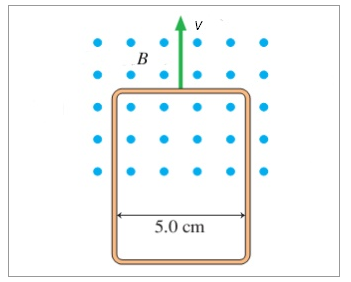# Problem: The loop in the figure is being pushed into the 3.00 T magnetic field at 10.0 m/s. (a) The resistance of the loop is 2.46 Ω. What is the magnitude of the current in the loop? (b) What is the direction of the current in the loop?

###### FREE Expert Solution

Motional emf:

$\overline{){{\mathbf{\epsilon }}}_{\mathbf{m}\mathbf{o}\mathbf{t}}{\mathbf{=}}{\mathbf{v}}{\mathbf{B}}{\mathbf{L}}}$

Ohm's  law:

$\overline{){\mathbf{V}}{\mathbf{=}}{\mathbf{i}}{\mathbf{R}}}$

(a)

Combining motional emf and Ohm's law expressions:

79% (66 ratings)###### Problem Details

The loop in the figure is being pushed into the 3.00 T magnetic field at 10.0 m/s.

(a) The resistance of the loop is 2.46 Ω. What is the magnitude of the current in the loop?

(b) What is the direction of the current in the loop?